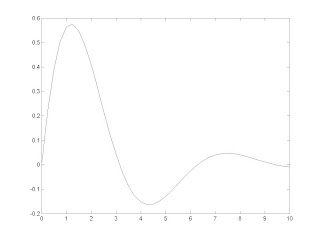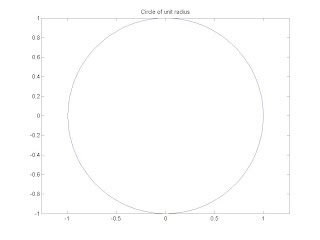# MATLAB programs, for 7th sem CSE studentsPrograms coded, compiled and tested by- Tushar kant verma, for CSVTU & CVRU students
Semester: VII Branch: Computer Science & Engg.
Subject: Soft Computing Lab. Code: 322721 (22)
Total Practical Periods:50
Total Marks in End Semester Exam: 40
1. WRITE MATLAB PROGRAM FOR FOLLOWING.
A) AREA = π r2 (USING ARITHMETIC OPERATOR).
B) 5e3 (USING EXPONENTIAL OPERATOR).

>>

C) y = Sin2 π/3 + Cos2π /3 (USING TRIGONOMETRY OPERATOR).

>>

D) y = Cos π/4 + i Sin π/4 (USING COMPLEX NUMBER).

>>

E) y= log10(106) (USING LOGARITHMS OPERATOR).

2. Compute y- coordinates of a STRAIGHT LINE y = mx + c, where slope of line m =0.5 , intercept c= -2 and x- coordinates : x = 0 to 10 for 0.5 increments.

Output: >> straightline Y- Co-ordinates are -2, -1.7500, -1.5000, -1.2500, -1, -0.7500, -0.5000, -0.2500, 0, 0.2500, 0.5000, 0.7500, 1, 1.2500, 1.5000, 1.7500, 2, 2.2500, 2.5000, 2.7500, 3
3. Create following vectors t with 10 elements 1 to 10.
a) x = t sin(t) [ A MULTIPLY VECTORS]

Output:
Multiple Vectors are
0.8415 1.8186 0.4234 -3.0272 -4.7946 -1.6765 4.5989 7.9149 3.7091 -5.4402
b) y = (t-1) / (t+1) [ A DIVIDE VECTORS]

Output:
Divide Vectors are
0 0.3333 0.5000 0.6000 0.6667 0.7143 0.7500 0.7778 0.8000 0.8182
c) z = [sin(t2)/ (t2)] [ A EXPONENTIAL VECTORS]

Output:
EXPONENTIAL VECTORS
0.8415 -0.1892 0.0458 -0.0180 -0.0053 -0.0275 -0.0195 0.0144 -0.0078 -0.0051
4. PLOT y = Sin x where 0 <= x <= 6.5.

Output:5. PLOT y = e-0.4x Sin x where 0 <= x <= 10.

Output:6. Write a script file to draw a unit circle.

Output:
>> prgcircle7. Write a function factorial to compute the factorial n! for any integer n.

Output:
Enter the number now 5
120
8. Write a function factorial to compute the factorial n! using RECURSION for any integer n.

9. Write a function file crossprod to compute the cross product of two vectors u and v.

Output:w1 = 0 0 1
w2 = 0 -1 0

10. Write a function to compute the geometric series
1 + r + r2 + r3 + ………+ rn for given r and n.

Note: To run script, do as follows:
Write and save the file under the name ‘Program_Name.m‘.

Now go back to the MATLAB command window and type the following command to execute the script file.

>>Program_Name